# Using Similar Figures

In earlier lessons, you learned that similar figures are figures that have the same shape — they have congruent corresponding angles and proportional corresponding sides. You learned to set up a proportion to find the missing side lengths. When two polygons are similar, the symbol ~ is used. When you know that polygons are similar, this allows you to find unknown lengths and angle measures. In this lesson, you will learn that the ratio of the lengths of corresponding sides in similar figures is sometimes called their scale factor.

## Investigate

When you compare similar shapes to find their scale factor, it is often easier to put the larger side first in the ratio. Scale factors can be expressed as a ratio or as a percent. Take a look at the following examples.

### Example 1Look at the triangles. We can see that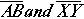are corresponding sides. Therefore, the scale factor is: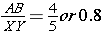Remember that when you compare similar triangles to find their scale factor, you sometimes put the larger side first in the ratio, so using the same triangles, you could say that the scale factor is:The scale factors for the triangles above could also be written as 80% or 125%. This simply means that one triangle is 80% as large as the other, or that one triangle is 125% larger than the other.

The best strategy for getting the order correct is to writewhen you are not sure how the question is to be answered.

### Example 2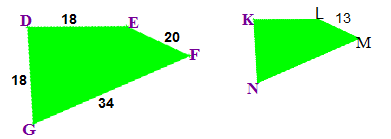These quadrilaterals are similar. Find their scale factor.

Remember to apply the definition of scale factor and use division.

Find a pair of corresponding side lengths. All lengths are given in DEFG, but onlyhas its length given in KLMN. Use the pair.

Calculate the scale factor:Solution: The scale factor is 65%

## Investigate

The Perimeter Rule for Scale Factor states that if similar figures have scale factor r, then the perimeters of those figures are in ratio r. Let’s see exactly what this means.

### Example 1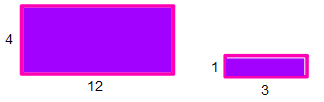Look at the rectangles to the left.

Apply the definition of the perimeter rule for scale factor for the rectangles.

First, find the scale factor, using one of the pairs of corresponding sides.. The scale factor is 4.

Next, find the perimeter of the small rectangle.

2(l) + 2(w) = 2(1) + 2(3) = 2 + 6 = 8

Next, multiply the perimeter by the scale factor.

8 * 4 = 32

The perimeter of the large rectangle is 32.

You can check this strategy by finding the length of each side and getting the perimeter of each rectangle. It works every time!

### Example 2

Let’s try this with a triangle.

A right triangle has sides of 3 cm, 4 cm, and 5 cm. The perimeter of this triangle would be 3 + 4 + 5 = 12. Can you find the perimeter of a triangle with a scale factor of 150%?

Apply the rule for finding perimeter of similar figures. If the perimeter of the original triangle is 12 and the scale factor is 150%, just multiply 12 by 1.5.

12 * 1.5 = 18. The perimeter of the new triangle would be 18. If you were asked to find the sides of the new triangle:

Start with the original triangle side lengths and multiply each one by 150%.

3 * 1.5 = 4.5

4 * 1.5 = 6

5 * 1.5 = 7.5

The side lengths of the new triangle would be 4.5 cm, 6 cm, and 7.5 cm. If you add these sides together, the perimeter would be 18 cm.

## Investigate

The area rule for scale factor states that if similar figures have scale factor r, then the areas of those figures are in ratio.

### Example 1Look at the rectangles to the left.

Use the corresponding sides to determine the scale factor:. The scale factor is 4.

Next, find the area of the small rectangle.Next, square the scale factor and multiply the area of the small rectangle by.

3 * 16 = 48

The area of the large rectangle is.

You can check this strategy by finding the length of each side of the rectangle and getting the area – It works every time!

### Example 2

A triangle has area of 20 sq. ft. A similar triangle is constructed using a 10% scale factor. What is the area of the new triangle?

1. Apply the area rule.
2. The triangles have scale factor r = 10% or 0.1
3. Square the scale factor: 0.1 * 0.1 = 0.01
4. Multiply the scale factor by the area of the original triangle.
5. 20 * 0.01 = 0.2 sq. ft.
6. The new triangle has an area of 0.2 square feet.

### Example 3

A rectangle has an area of 30 feet. A similar rectangle has an area of 270 feet. What is the scale factor?

1. First, divide 270 by 30 = 9.
2. Then, find the square root of that number.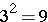.
3. Therefore, the scale factor of these two rectangles is 3.

Let’s quickly review these strategies and rules:

1. To get the scale factor, simply write the ratio of the two figures as New over Original. (unless otherwise specified).
2. To get the perimeter ratio, multiply the perimeter by the scale factor.
3. To get the area ratio, multiply the area by the scale factor squared (for 2 dimensional figures).
4. To find the scale factor from the area, work backwards – find the area ratio and UNsquare it (find its square root).

## Similar Figures Practice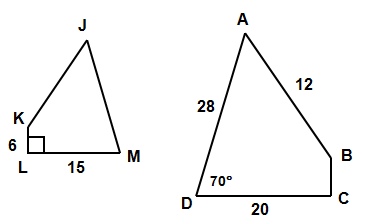Quadrilateral ABCD ~ JKLM.  Complete each of the following:

1. The scale factor (%) of ABCD to JKLM is _____

2. BC = ______

3.  JM = ______

4.  JK = ______

5.  The ratio of the perimeter of ABCD to the perimeter of JKLM = ______

6.  The ratio of the area of ABCD to the area of JKLM = ________

(source)